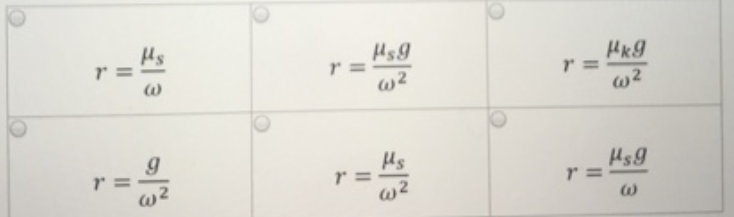# Problem: A penny is placed a distance r from the center of a record spinning at ω = 90π rad/min. The coefficient of static friction between the penny and the record is μs.= 0.11 on the horizontal plane. Part (a) Select an expression for the maximum distance, r, the penny can be placed from the center and not move.  Part (b) What is the distance, r in meters?

###### FREE Expert Solution

Part (a)

Equilibrium of forces:

82% (304 ratings)###### Problem Details

A penny is placed a distance r from the center of a record spinning at ω = 90π rad/min. The coefficient of static friction between the penny and the record is μs.= 0.11 on the horizontal plane.

Part (a) Select an expression for the maximum distance, r, the penny can be placed from the center and not move.Part (b) What is the distance, r in meters?

Frequently Asked Questions

What scientific concept do you need to know in order to solve this problem?

Our tutors have indicated that to solve this problem you will need to apply the Flat Curves concept. You can view video lessons to learn Flat Curves. Or if you need more Flat Curves practice, you can also practice Flat Curves practice problems.

What professor is this problem relevant for?

Based on our data, we think this problem is relevant for Professor Foster's class at VCU.